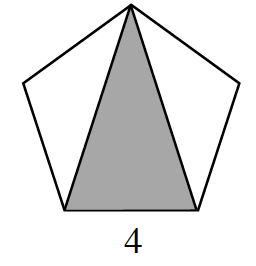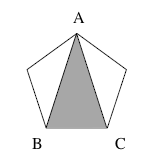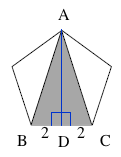### Home > GC > Chapter 12 > Lesson 12.2.3 > Problem12-77

12-77.

Find the area of the shaded region of the regular pentagon at right. Show all work.Label the shaded region as $ΔABC$.

The measure of each angle in a pentagon is equal to $\frac{180(5 \cdot 2)}{5} = 180^\circ$.

Therefore, the measure of $\angle BAC$ is equal to $\frac{108}{3} = 36^\circ$

Divide $\Delta ABC$ into two triangles. Use a trigonometric ratio to solve for $AD$.

Solve for the length of AD:  $\tan 18^\circ = \frac{2}{AD}$

Once you know the length of AD, use the formula (1/2)(Base)(Height) to find the area of the shaded triangle.